How Can We Help?

Search for answers or browse our knowledge base.

Geometry Constraint

Geometry constraint can keep the certain relationship between the objects.

Geometry constraint includes parallel, perpendicular, horizontal, vertical, tangent, concentric, coincident, equal, collinear, symmetrical, fixed constraints and same size. The valid entities for geometry constraint includes line, circle (arc), point.

Command: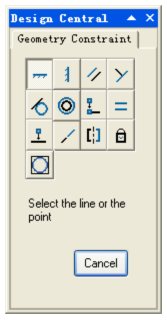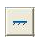Horizontal: Make the line keep parallel with X axis

Valid Objects: Line, polyline subobjects and two valid constraint points.

Steps:

1.Select.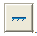2.Select the line or 2 points.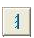Vertical: Make
the line keep parallel with Y axis.

Valid Objects: Line,polyline subobjects and two valid constraint points.

Steps:

1.Select.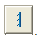2.Select the line or 2 points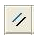Parallel：Make two lines keep parallel with each other.

Valid Objects: Line and polyline subobjects.

Steps:

1.Select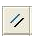in Design Central

2.Select the first line.

3.Select the second line.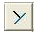Perpendicular: Make two lines keep Perpendicular with each other.

Valid Objects: Line and polyline subobjects.

Steps:

1.Select.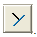2.Select the first line.

3.Select the second line.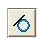Tangent: make two curves keep tangent with each other.

Valid Objects ：Line, polyline subobjects, circle (arc) .

Steps:

1.Select.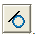2.Select the first curve.

3.Select the second curve.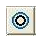Concentric: Make two circles(arc) keep the same center point.

Valid Objects: Circle (arc), ellipse.

Steps:

1.Select.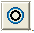2.Select the first object.

3.Select the second object.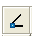Coincident: Make the two points keep coincide with each other.

Valid Objects: Line, polyline subobject, circle (arc), point.

Steps:

1.Select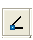.

2.Select the first point.

3.Select the second point.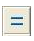Equal: Make two circles or arcs keep equal in radius; or Make two lines keep equal in length.

Valid Objects: Line, polyline subobject, circle (arc), point.

Steps:

1.Select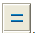.

2.Select the first curve.

3.Select the second curve.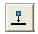Collinear: Make
the point always online (or on the extension cord).

Valid Objects: Line, polyline subobject, circle (arc), point.

Steps:

1.Select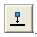.

2.Select the point.

3.Select the curve.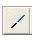Collinear: Make two lines along the same line.

Valid Objects: Line, polyline subobject

Steps:

1.Select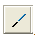.

2.Select the first line.

3.Select the second line.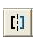symmetrical: Make two objects keep symmetric.

Valid Objects: Line, polyline subobject, circle (arc).

Steps:

1.Select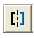.

2.Select the first point.

3.Select the second point.

4.Select the symmetric axis line.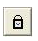Fix: Lock a point of object.

Valid Objects: Line, polyline subobject, circle (arc).

Steps:

1.Select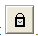.

2.Select the point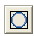Same size: Select two objects and make them to keep same size.

Valid Objects: all objects

Steps:

1.Select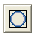2.Select the object of the transform.

3.Select the reference object.